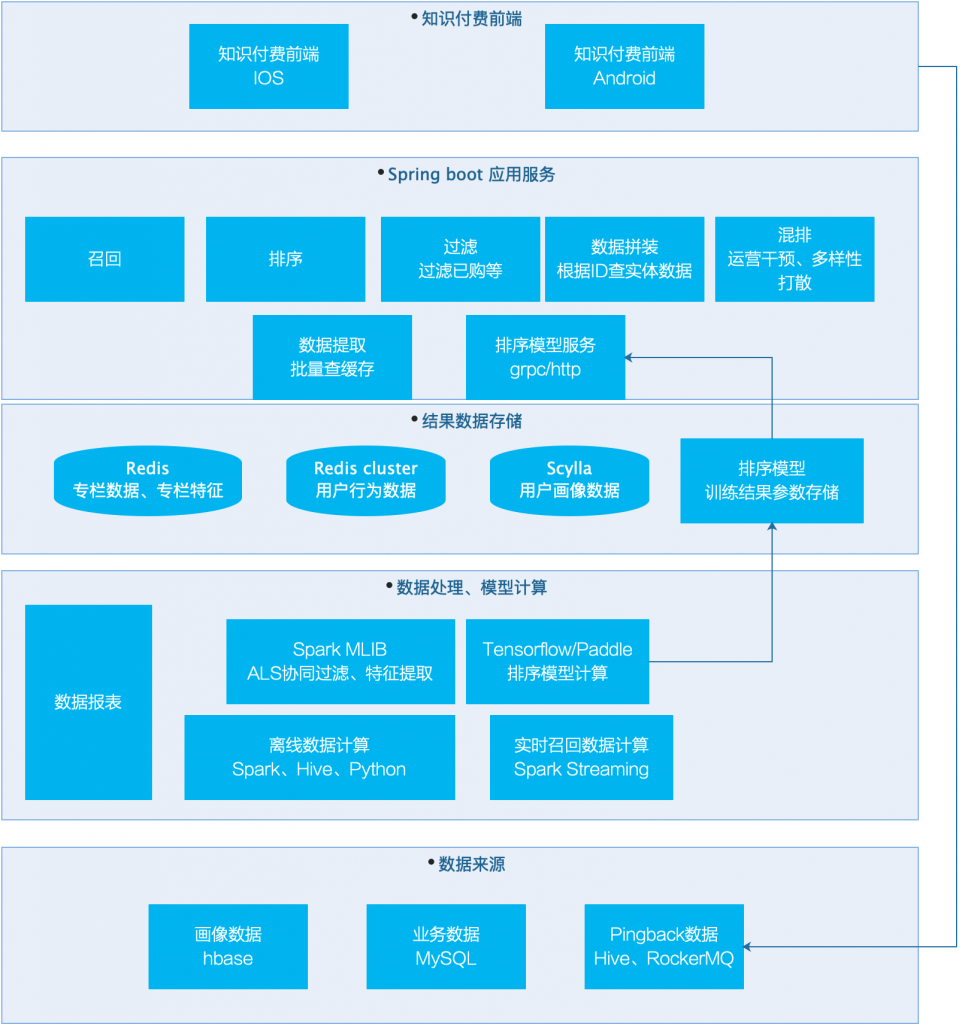## 推荐系统实现相似推荐的流程和资源

1、jieba中文分词库：

https://github.com/fxsjy/jieba

2、spark word2vec计算：

http://spark.apache.org/docs/latest/ml-features.html#word2vec

3、腾讯开源800万word2vec数据：

https://ai.tencent.com/ailab/nlp/embedding.html

4、Scipy的相似度计算函数：

https://docs.scipy.org/doc/scipy-0.14.0/reference/generated/scipy.spatial.distance.cosine.html

5、相似度近邻搜索优化算法LSH局部敏感哈希

http://spark.apache.org/docs/latest/ml-features.html#locality-sensitive-hashing

## 推荐系统：实现文章相似推荐的简单实例

1、将CSV加载到pandas.pd

2、提取其中的标题、题材分类、导演、演员、情节描述4个字段；

3、将单词都变小写，人名中的空格合并(英文才需要这样)；

4、题材分类、导演、演员这几个特征都是结构化的不需要处理；而标题、情节描述这类字段是长段文本，使用nltk库做关键词提取(如果是中文可以用jieba分词库也有关键词提取功能)

5、将第四步骤的分类、导演、演员、关键词列表，合并到一个词列表(这一处理其实暗含了分类、导演、演员三个特征和关键词一样重要，没有做加权处理)

6、使用CountVectorizer做每个词语的计数，得到了每个文章的向量；

7、使用sklearn的cosin做笛卡尔积的相似度计算；

8、计算结果是一个二维矩阵，按行查询某一个文章的推荐结果，按相似度值排序得到最相似的文章

1、全流程用pandas运行，尤其是for each row，做单个列的各种map计算；

2、计算相似度时使用了多个特征，包括Title,Genre,Director,Actors,Plot，统一成一个bag of words参与计算

3、使用from sklearn.metrics.pairwise import cosine_similarity用于相似度计算；

4、使用from sklearn.feature_extraction.text import CountVectorizer用于单词计数；

5、使用from rake_nltk import Rake用于关键词提取；

```import pandas as pd
from rake_nltk import Rake
import numpy as np
from sklearn.metrics.pairwise import cosine_similarity
from sklearn.feature_extraction.text import CountVectorizer```

pandas的dataframe中，直接替换一列的语法：

`df['Director'] = df['Director'].map(lambda x: x.split(' '))`

pd按行以此处理某个列的方法：

```for index, row in df.iterrows():
row['Actors'] = [x.lower().replace(' ','') for x in row['Actors']]
row['Director'] = ''.join(row['Director']).lower()```

pd.df删除某一列的方法：

`df.drop(columns = ['Plot'], inplace = True)`

pd.df从columns中提取一列作为index的方法：

`df.set_index('Title', inplace = True)`

```df['bag_of_words'] = ''
columns = df.columns
for index, row in df.iterrows():
words = ''
for col in columns:
if col != 'Director':
words = words + ' '.join(row[col])+ ' '
else:
words = words + row[col]+ ' '
row['bag_of_words'] = words

df.drop(columns = [col for col in df.columns if col!= 'bag_of_words'], inplace = True)```

sklearn使用词计数的调用：

```count = CountVectorizer()
count_matrix = count.fit_transform(df['bag_of_words'])```

sklearn实现矩阵相似度计算的方法：

```# generating the cosine similarity matrix
cosine_sim = cosine_similarity(count_matrix, count_matrix)
```

I decided to use `CountVectorizer` rather than `TfIdfVectorizer` for one simple reason: I need a simple frequency counter for each word in my `bag_of_words` column. Tf-Idf tends to give less importance to the words that are more present in the entire corpus (our whole column, in this case) which is not what we want for this application, because every word is important to detect similarity!

1、标题、简介，提取关键词后，可以查询业界的word2vec做向量扩展，这样能实现恰恰和伦巴舞这类词语的相似度度量，直接的关键词查询是得不到这样的信息；

2、分类、导演、演员这三个特征，需要和描述得到的关键词区分开，可以用加权的方法进行，按照产品的需求，加重分类、导演的相似度权重，降低演员、关键词的权重等，如果需要可以从点击率等出发，用模型计算这些权重；

## Spark使用word2vec训练item2vec实现内容相关推荐

spark版本：2.3.1，开发语言为JAVA

## 几大步骤

1. 读取查看、点击、播放等行为数据，我用的是播放数据；
2. 数据整理成(userid, itemid, playcnt)的形式，这个数据可能是聚合N天得到的；
3. 过滤掉playcnt为小于3的数据，我把这些过滤掉，觉得这个数据没有贡献；
4. 按照userid聚合，得到(userid, list(itemid))的形式；
5. 训练word2vec；
6. 导出model.vectors()，里面包括word和对应的向量vector，其中word其实就是itemid
7. crossjoin计算两两相似度，取相似度TOP N；
8. 将结果存入mysql，后续可以加载到REDIS实现实时相似推荐；

## 代码实现

```Dataset<Row> playDatas = spark.sql(
"select user_id, item_id, play_cnt " +
"from hive_play_table group by user_id, item_id");```

```playDatas = playDatas
// 删除掉只播放3次以下的数据
.filter("play_cnt>2")
// 按userid聚合
.groupBy("user_id")
.agg(collect_list("item_id").as("item_ids"))
// 至少操作过2个元素
.where(size(col("item_ids")).geq(2));```

```Word2Vec word2Vec = new Word2Vec()
.setInputCol("item_ids")
.setOutputCol("word2vec_result")
.setVectorSize(50)
.setMinCount(0)
.setMaxIter(50)
.setSeed(123);

Word2VecModel word2VecModel = word2Vec.fit(playDatas);```

```Dataset<Row> vectorsA = word2VecModel
.getVectors()
.select(
col("word").as("itemIdA"),
col("vector").as("vectorA"));

Dataset<Row> vectorsB = word2VecModel
.getVectors()
.select(
col("word").as("itemIdB"),
col("vector").as("vectorB"));

// self cross join
Dataset<Row> crossDatas = vectorsA.crossJoin(vectorsB);```

```spark.udf().register(
"vectorCosinSim",
new UDF2<Vector, Vector, Double>() {
@Override
public Double call(Vector vectora, Vector vectorb) throws Exception {
return SimilarityUtils.cosineSimilarity(vectora, vectorb);
}
},
DataTypes.DoubleType
);```

```public static double cosineSimilarity(Vector featuresLeft, Vector featuresRight) {
double[] dataLeft = featuresLeft.toArray();
List<Float> lista = new ArrayList<>();
if (dataLeft.length > 0) {
for (double d : dataLeft) {
}
}

double[] dataRight = featuresRight.toArray();
List<Float> listb = new ArrayList<>();
if (dataRight.length > 0) {
for (double d : dataRight) {
}
}

return cosineSimilarity(lista, listb);
}```

```crossDatas = crossDatas
.withColumn(
"cosineSimilarity", callUDF(
"vectorCosinSim", col("vectorA"), col("vectorB")))
.select("itemIdA", "itemIdB", "cosineSimilarity")
.filter(col("itemIdA").notEqual(col("itemIdB")));
```

```// 按照相似度倒序排列取TOP 300
WindowSpec windowSpec = Window.partitionBy("itemIdA").orderBy(col("cosineSimilarity").desc());
crossDatas = crossDatas
.withColumn("simRank", rank().over(windowSpec))
.where(col("simRank").leq(200));```

```crossDatas = crossDatas
.groupBy("itemIdA")
.agg(
collect_list("cosineSimilarity").as("columnSims"),
collect_list("itemIdB").as("itemIds")
).select(
col("itemIdA").as("item_id").cast(DataTypes.LongType),
col("columnSims").as("column_sims").cast(DataTypes.StringType),
col("itemIds").as("item_ids").cast(DataTypes.StringType)
);```

```crossDatas.write().mode(SaveMode.Overwrite).jdbc(
MysqlConfig.ONLINE_MYSQL_MASTER_URL,
"item2vec_sims",
MysqlConfig.getOnlineProperties()
);```

## 推荐系统：怎样实现内容相似推荐

1、获取内容数据，比如标题、关键字、分类、甚至全文本；

2、对内容数据做中文分词；

3、提取内容数据的关键词；

4、将关键词映射成数字向量；

• 使用one hot方法映射成向量
• 自己训练word2vec密度向量；
• 使用业界的训练好的word2vec向量

5、计算文档粒度的数字向量；

6、计算文档之间的相似度；

cosin算法很简单，java、python自己实现就可以，也可用https://scikit-learn.org或者http://surpriselib.com/中的相似度计算模块直接调用实现。

7、将文档的相似度关系存入mysql/redis等缓存；

8、当页面展示一个内容的时候，提取相似推荐进行展示；

## 1、表现形式• 个性化分群运营：根据画像做用户分群，按群推荐课程；
• 猜你喜欢：个性化课程推荐流；
• 相关推荐：课程页面内的相关推荐；
• 买了又买：购买成功页的课程推荐；

## 2、系统架构• 数据处理：使用spark、hive、python，从mysql备库、hbase、pingback的hive表和实时流提取数据，实现关联、清洗、映射特征等处理，其中spark streaming从pingback的rockermq准实时提取用户行为，实现10秒钟用户个性化推荐列表的更新；
• 结果数据存储：这里涉及比较多的考虑，主要是从数据量、查询响应时间、数据结构支持度等方面考虑：
• 对于item本身实体数据、item相似度列表等数据，使用redis存储，因为redis cluster不能够批量读取，但是这里却需要批量读取；
• 对于用户行为实时数据，因为需要大数据量存储，并且需要丰富的数据结构支持，选用redis cluster；
• 对于用户画像数据、自己提取的用户标签数据，数据量大，只需要根据用户ID提取，所以选用scylla；
• 召回、排序、已购等步骤，都是id在参与计算，等最终返回的时候，才查询实体业务数据；
• 模型排序服务使用grpc/brpc/http提供服务给应用服务调用；
• 应用服务同时需要支持AB Test的分桶，以及参数的返回；
• 前端请求：前端只进行非常短暂的缓存（防止恶意请求），重要的是需要搞定pingback的埋点投递，投递中需要设定ab test的参数

• 当前系统在QPS高峰是耗时150MS左右，时间耗费在实体数据拼装阶段，后续进行优化；
• 相关推荐的导流效果很好，后续进行多种实验进行效果对比；
• 排序模型的线上化，通过AB测试进行效果对比；